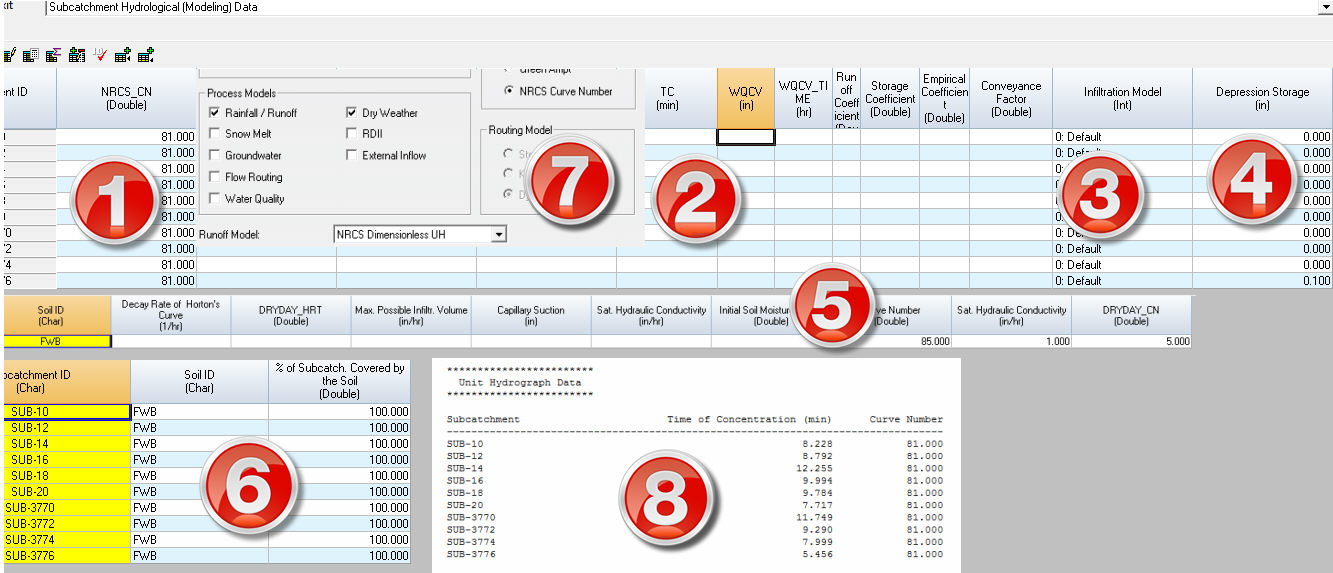#INFOSWMM

# Rules for using the NRCS (SCS) Dimensionless Unit Hydrograph Method in InfoSWMM and InfoSWMM SA

Rules for using the NRCS (SCS) Dimensionless Unit Hydrograph Method in InfoSWMM and InfoSWMM SA

A unit hydrograph is defined as the direct runoff hydrograph resulting from a unit depth of excess (effective) rainfall produced by a storm of uniform intensity and specified duration. Unit hydrographs could be natural or synthetic. Natural unit hydrographs are derived from observed data, whereas synthetic unit hydrographs are generated following empirical techniques based on watershed parameters and storm characteristics to simulate the natural unit hydrograph. In addition to the rational formula, H2OCalc computes peak flow from a watershed using the NRCS (SCS) dimensionless unit hydrograph.

The NRCS dimensionless unit hydrograph, graphically descried below, is widely used in practice. To generate a tr-hour unit hydrograph for a watershed, time to peak (Tp) and the peak flow rate (Qp) are determined using watershed characteristics.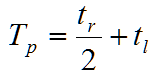where tr is duration of effective rainfall, and tl is lag time of the watershed. Lag time represents the time from the center of mass of effective rainfall to the time to peak of a unit hydrograph. In other words, lag time is a delay in time, after a brief rain over a watershed, before the runoff reaches its peak. The lag time can either be specified by the user, or can be calculated by the model using the following SCS equation.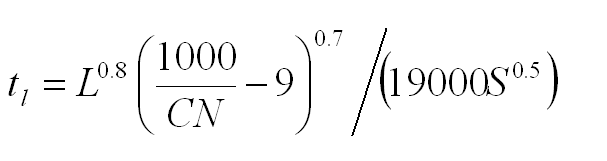where tl = lag time of the watershed (hr).

L = hydraulic length of the watershed (ft). This refers to travel distance of water from the most upstream location of the watershed to the point where the unit hydrograph is derived.

CN = the SCS curve number. This is a measure of runoff generating capacity of a watershed, and it depends on the soil, the antecedent moisture condition, the cover, and the hydrologic conditions of the watershed. Recommended CN values are given in the following table for urban areas (USDA 1986). The SCS suggests the CN values for the above equation to be within 50 and 95.

S = average slope of the watershed.

The peak flow rate is calculated as:where Qp = peak flow rate (ft3/s).

A = area of the watershed, in square miles, draining to the location of the unit hydrograph.

Tp = time to peak of the unit hydrograph in hours.

Once Tp and Qp are known, actual time and flow rate ordinates of the tr-hour unit hydrograph are determined by multiplying the dimensionless time (T/Tp) and the dimensionless flow rate ordinates (Q/Qp) by Tp and Qp, respectively.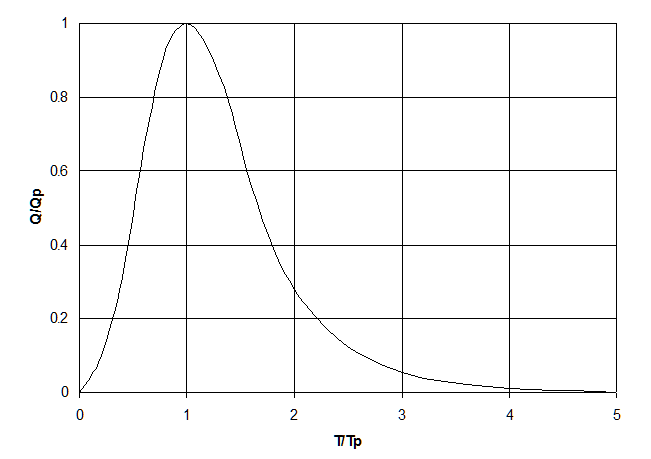NRCS Dimensionless Unit Hydrograph

Storm hydrograph could be computed using the unit hydrograph and a given precipitation pattern. The precipitation could be a design precipitation or actual (i.e., historical precipitation). A design precipitation may be specified in the form of rainfall depth over 24-hr duration. Distribution of the rainfall depth across the 24-hour can be estimated using the SCS (NRCS) rainfall types (i.e. Type I, Type IA, Type II and Type III) shown in the following figure.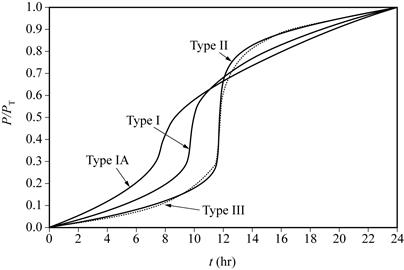SCS (NRCS) Rainfall Types (Source Nicklow et al., 2006)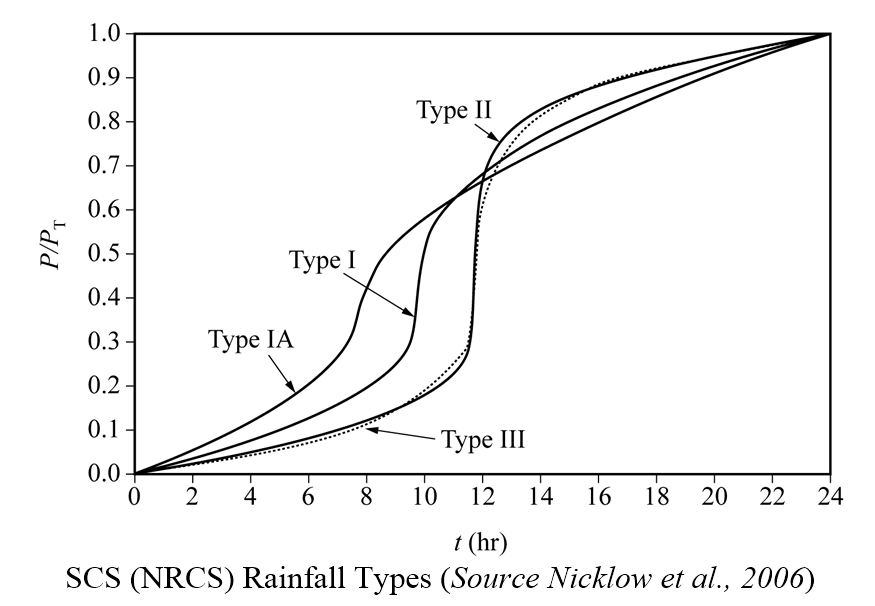Rules for Entering the SCS CN in the Dialogs

The rules are listed below but in paragraph style. The program will use the TC and SCS CN from the Subcatchment table to calculate the UH from the Rainfall Excess. The Rainfall Excess is calculated from the Soils DB Table using the Soils Coverage in the Infiltration Tables. If you do not have a Soils Table and an Infiltration Coverage Table the program will make the Rainfall Excess equal to the Rainfall and you will not have any Infiltration Losses. The default value for Initial abstraction from IA = 0.2 * (1000/CN -10)

Rule 1. The Column NRCS CN in the Subcatchment Table is the CN used in the Unit Hydrograph Routing

Rule 2. If the Column TC in the Subcatchment Table is Blank then the program will estimate TC in minutes from the Slope and other parameters

Rule 3. Use Default as the Infiltration Model for consistency in the Subcatchment Table.

Rule 4. If the Depression Storage for the UH is 0.0 then the initial abstraction for the UH Is calculated from the CN in the Subcatchment Table

Rule 5. The CN for the Soil is entered in the Soil DB Table

Rule 6. The Soil coverage for each Subcatchment is entered in the Infiltration DB Table and the program will calculate the weighted by area infiltration CN

Rule 7. Use a Runoff Model of NRSCS UH and an Infiltration model of CN in Run Manager

Rule 8. The TC and UH CN are listed in the output report file of InfoSWMM H2OMap SWMM